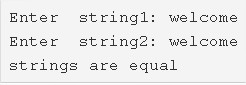# C Program To Compare Two Strings – 3 Easy Ways | C Programs

C program to compare two strings – In this specific article, we will describe the various ways to compare two different strings in C programming.

Suitable examples and sample programs have also been added so that you can understand the whole thing very clearly. The compiler has also been added with which you can execute it yourself.

The ways used in this particular piece are as follows:

• Using Standard Method
• Using Function
• Using Recursion
• Using String Library Function

A string is nothing but an array of characters. The value of a string is determined by the terminating character. Its value is considered to be 0.In the photo uploaded above, an example is showcased. A string needs to be entered first.

The string that is entered in the first case is as follows:

string1: welcome

Then, enter the second string that you want to compare with the first one.

string2: welcome

As you can see, both the strings are absolutely equal in nature.

Thus, the numerous methods to do the same in C programming are as follows:

## Using Standard Method

1. Read the entered strings s1 and s2 using gets(String) function and initialize to s1,s2.

2) First, check the lengths of two strings. If lengths of two strings are equal then only we can compare the strings. Compare each element of string s1 with each element of string s2 as follows.

a) for loop iterates with the structure for(i=0;s2[i]!=’\0′;i++)

If the element of string s1 is equal to the element of string s2 then increase the c value by 1. Repeat this step until the last character of s2 becomes null.

b) If c value is equal to i value,i.e each character of s1 is equal to each character of s2. Then print both the strings are equal. Otherwise print both the strings are not equal.

3) If the lengths of the two strings are not equal then print both the strings are not equal.

Output:

### Using Function

1. The main() function calls the stringcompare(char *s1,char *s2) function to compare the two strings.

2) If the length of the two strings is equal then,

a) compare each character of the string s1 with each character of the string s2 using for loop with the structure for(i=0;s2[i];i++).

b) If a character of string s1 is equal to a character of string s2 then increase the c value. Repeat till the last element of the string s1.

If c is equal to i then print both the strings are equal.

3) If the lengths of two strings are not equal then print two strings are not equal.

Output:

#### Using Recursion

1. The function stringcompare() calculates the lengths of two strings as l1=strlen(s1) and l2=strlen(s2).

2) If the length of string s1 is not equal to the length of string s2 then the function stringcompare() returns 0.

3) If two strings lengths are equal,

a) Then compare s1[i] with s2[i],if s1[i] equal to s2[i] then increase c value,i value.

b) The function calls itself as stringcompare(s1,s2).It calls itself until i<l1 becomes false.

4) If c is equal to i then this function returns 1 otherwise it returns 0.

5) If c=1 then main function prints two strings are equal,if c=0 it prints two strings are not equal.

Output:

##### Using String Library Function
1. The function strcmp(String1, String2) is the string library function to compare two strings. If both the strings are equal then it returns 1 otherwise it returns 0.

2) The function strcmp(String1, String2) is available in the string.h header file.

Output:

x

## C Program To Print Number Of Days In A Month | Java Tutoring

C program to input the month number and print the number of days in that ...